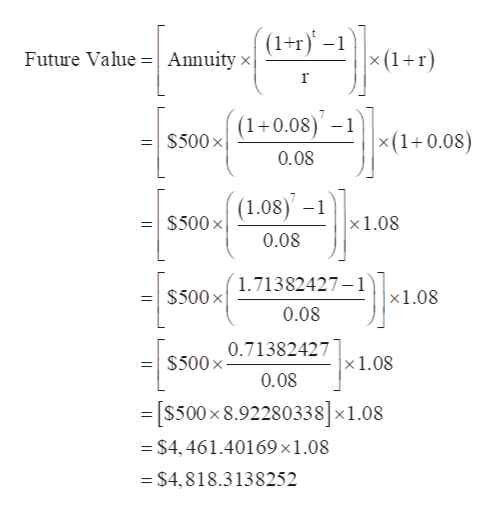# The future value of \$500 to be received at the beginning of each year for seven years ata discount rate of 8 percent is ? .

Question
2 views

The future value of \$500 to be received at the beginning of each year for seven years at
a discount rate of 8 percent is ? .

check_circle

Step 1

Annuity = \$500

Time Period (t) = 7 Years

Discount Rate (r) = 8%

...help_outlineImage Transcriptionclose(1+r)' –1 × (1+r) Future Value =| Annuity × (1+0.08)' – 1 \$500 x| ×(1+0.08) %3D 0.08 (1.08)' –1 = \$500 x x1.08 0.08 1.71382427–1 =| \$500 x x1.08 0.08 0.71382427 \$500 x- ×1.08 0.08 =[s500 x 8.92280338]×1.08 = \$4, 461.40169×1.08 = \$4,818.3138252 fullscreen

### Want to see the full answer?

See Solution

#### Want to see this answer and more?

Solutions are written by subject experts who are available 24/7. Questions are typically answered within 1 hour.*

See Solution
*Response times may vary by subject and question.
Tagged in